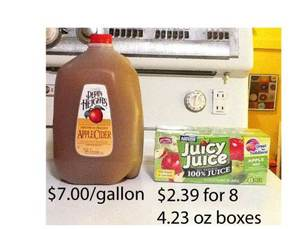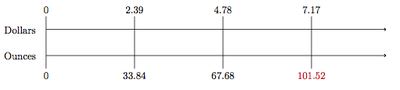# Cider versus Juice - Variation 2

Alignments to Content Standards: 7.RP.A.1 7.RP.A.2.a 7.RP.A.2.b1. Assuming you like juice and cider equally, which product is the better deal?
2. Suppose the juice boxes go on sale for \$1.79 for the eight 4.23-ounce juice boxes, and the cider goes on sale for \$6.50 per gallon. Does this change your decision?

## IM Commentary

The goal of this task is to apply proportional reasoning to determine which of two ways of buying apple juice/cider is a better deal. The question is opened ended, allowing for a variety of approaches including

• Using a double number line
• Using a ratio table
• Setting up a proportion
• Scaling using estimation and mental math

This task is a second variation to 7.RP.A.1, 7.RP.A.2.b Cider Versus Juice - Variation 1, https://www.illustrativemathematics.org/tasks/1968. This version offers a less directed approach to one of the questions posed in that task.

This task could be implemented on its own as a handout for students, or, ideally, via the blog post which inspired it, which can be found at http://christopherdanielson.wordpress.com/2011/11/29/cider-price-rules/. The post could be displayed/distributed first to the whole class in order to generate a conversation prior to administering the task. This allows for students to connect with the real-world scenario they’re being put in, as well as to formulate their own guesses as to which product would be a better monetary buy and why.

Instructors may need to help students decipher the phrase "better deal". Students need to understand that we do not simply mean which is the cheapest as-is, but which is the cheapest per unit of volume of liquid. Students will also need to realize that not all needed information is given for this task, namely, the conversion rate between gallons and ounces. Instructors can either let students look this up themselves or have it available for them, but ideally students should come to the conclusion themselves that they need to know this rate in order to continue. The conversion rate is 128 fluid ounces per gallon. If students struggle with the distinction between ounces and fluid ounces, it could be a good opportunity to discuss mass versus volume measurements, and how to tell by context which one is appropriate to use.

Because the problem is open ended, instructors should encourage students to present or prove their findings in more than one way! This may help to get students thinking about double number lines, tables, and unit rates all together.

## Solution

1. The cider costs \$7.00 for a gallon. The juice cost is based in ounces, so we first find how many total ounces of juice comes in the boxes. There are 8 boxes, each containing 4.23 ounces, for a total of$8 \times 4.23 = 33.84$ounces of juice. There are 128 ounces in a gallon, and we use this fact to compare the cost of the cider to the cost of the juice. We can use a double number line as below for the price of the juice:Clearly, we see that we pay \$7.17 well before we reach 128 ounces of juice so the cider is cheaper (per gallon) than the juice. Alternatively, we can present the information in a ratio table:

 ​Amount of Juice, oz Cost of Juice, $0 0 33.84 2.39 67.68 4.78 101.52 7.17 We see the same results as we did in the number line, mainly that we pay \$7.17 for 101.52 ounces of juice, which is less than a gallon. We can also set up a proportion to calculate a unit rate:

$$\frac{2.39\text{ dollars}}{33.84 \text{ ounces}} = \frac{x \text{ dollars}}{1 \text{ gallon}}$$

and solving for $x$ gives us

$$\frac{2.39\text{ dollars}}{33.84 \text{ ounces}} \cdot \frac{128\text{ ounces}}{1\text{ gallon}} \approx \frac{9.04 \text{ dollars}}{1\text{ gallon}},$$

so $x\approx 9.04$, and the price of the juice is about \$9.04 per gallon. Compared with the \$7.00 per gallon price tag of the cider, the juice costs more.

Finally we could use mental math and estimation. The juice boxes are 4.39 ounces and 8 of them cost \$2.39. This is more expensive than 5 ounce juice boxes \$2$\frac{1}{3}$. There are 40 ounces of juice in each of these packages so three of them would be 120 ounces of juice for \$7. This is a smaller amount than the cider for the same price so the better buy is the cider. 2. To determine if the cider or the juice is the better buy with the new sale prices, we first show the number line diagram representation, where the red arrow indicates 128 ounces:Notice that buying three packages of the juice is less than 128 ounces and costs \$5.37, but buying four packages is more than 128 ounces and costs \$7.16. As we are comparing these costs with the cider, which costs \$6.50 for 128 ounces, this does not give us enough information as is. Finding the cost of 128 ounces of the juice can be done by scaling, for example, 135.36 by $\frac{128}{135.6}$ and then doing the same for \$7.16: this is similar to setting up a proportion which is shown below. Alternatively, we could calculate the unit rate for the number of ounces of juice that we can by for \$1 and then scale these values by 7. We show in the ratio table below how to use this idea of finding how much juice we can buy for \$1:  Amount of Juice, oz Cost of juice,$ 0 0 33.84 1.79 33.84 $\div$ 1.79 1 (33.84 $\div$ 1.79) $\times$ 6.5 $\approx$ 123 6.5

The ratio table shows that for \$6.50 we can buy about 123 ounces of juice, a little less than the 128 ounces of cider so the cider is a better buy. Using the proportion and unit rate approach, we have $$\frac{1.79\text{ dollars}}{33.84 \text{ ounces}} = \frac{x \text{ dollars}}{1 \text{ gallon}}$$ and solving for$x$gives us $$\frac{1.79\text{ dollars}}{33.84 \text{ ounces}} \cdot \frac{128\text{ ounces}}{1\text{ gallon}} \approx \frac{6.77 \text{ dollars}}{1\text{ gallon}},$$ so$x\approx 6.77$, and the price of the juice is \$6.77 per gallon. Compared with the \\$6.50 per gallon price tag of the cider, the juice still costs more.